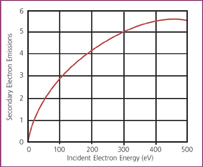# Gain Theory

SECONDARY ELECTRON EMISSIONThe basic physical process that allows an electron multiplier to operate is called secondary electron emission. When a charged or neutral particle, an ion or an electron, strikes a surface it can cause electrons associated with the outer layers of atoms to be released. The number of secondary electrons released depends on the type of incident primary particle, its angle, energy and characteristics of the incident surface.

In general there are two basic forms of electron multipliers that are commonly used in mass spectrometry; the discrete-dynode electron multiplier, and the continuous-dynode electron multiplier (often referred to as a channel electron multiplier or CEM). ETP electron multipliers are of the discrete-dynode type.

A typical discrete-dynode electron multiplier has between 12 and 24 dynodes and is used with an operating gain of between 104 and 108, depending on the application.  In GC-MS applications, for example, the electron multiplier is typically operated in analogue mode with a gain of around 105.  For a new electron multiplier this gain is typically achieved with an applied high voltage of ~1400 volts.

The electrical current, I, seen at the output of the multiplier is determined by:

I = q N G

-where q is the charge on an electron, N is the number of ions per second being detected, and G is the gain of the multiplier.

So the amount of electrical current taken from the multiplier output is directly related to the number of ions detected, and on the operating gain of the multiplier.